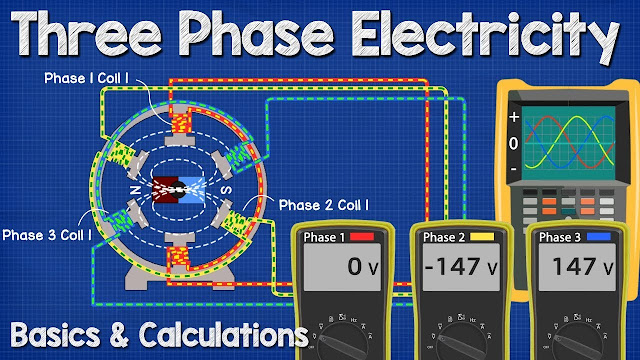Three Phase Electricity Basics and Calculations electrical engineering

# Three Phase Electricity Basics and Calculations electrical engineeringThree Phase Electricity Basics and Calculations electrical engineering

Three-phase electric power is a common method of alternating current electric power generation, transmission, and distribution.It is a type of polyphase system and is the most common method used by electrical grids worldwide to transfer power. It is also used to power large motors and other heavy loads.
A three-wire three-phase circuit is usually more economical than an equivalent two-wire single-phase circuit at the same line to ground voltage because it uses less conductor material to transmit a given amount of electrical power. Polyphase power systems were independently invented by Galileo Ferraris, Mikhail Dolivo-Dobrovolsky, Jonas Wenström, John Hopkinson and Nikola Tesla in the late 1880s.

In this video we learn how three phase electricity works from the basics. We explain how three phase electricity is generated, how the voltages vary, how to calculate the voltages and vrms, what frequency, hertz and cycle mean and how we get two different voltages from a three phase supply. We look at wye and delta, transformers and generators. We start with a simple single phase AC generator and then add in a second and third phase to under stand its working principle.

Related topics: